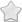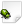CLOSED  Crash report #1537  -  [D5929FB1] Thread::entryPoint() basic_string.h:2290
Posted Apr 16, 2021 - updated Aug 20, 2021   Shortlink: http://arx.vg/1537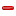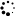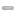0 Votes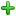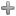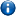This issue is a duplicate of issue #1094 - "[F4AE7D16] Thread::entryPoint() Thread.cpp:144"This issue has been closed with status "Not a bug" and resolution "DUPLICATE".
Issue details
• Type of issue
Crash report
• Status
 Not a bug
• Assigned to
Not assigned to anyone
• Type of bug
Not triaged
• Likelihood
Not triaged
• Effect
Not triaged
• Posted by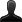CrashBot
• Owned by
Not owned by anyone
• Estimated time
Not estimated
• Category
Not determined
• Resolution
DUPLICATE
• Priority
Not determined
• Reproducability
Not determined
• Severity
Not determined
• Targetted for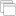Not determined
• OS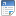Linux
• ArchitectureOther
• Fixed inNot determined
Issue description
Illegal storage access: address not mapped to object

``` Instruction address: 0x102de4
Memory accessed: 0xffffffff81000020
Stack pointer: 0xe2efee38
Frame pointer: 0xe2efef7c
```

Callstack:
``` arx!0x10be2c
??!0xf72ae130
arx!0x102de4
```

GDB stack trace:
``` [New LWP 1376]
[New LWP 1377]
[New LWP 1378]
[New LWP 1379]
Id   Target Id                                  Frame
2    Thread 0xf4693440 (LWP 1376) "threaded-ml" __GI___poll (timeout=121, nfds=3, fds=0x17cd878) at ../sysdeps/unix/sysv/linux/poll.c:29
3    Thread 0xe3e92440 (LWP 1377) "arx"         futex_wait_cancelable (private=0, expected=0, futex_word=0x16050dc) at ../sysdeps/unix/sysv/linux/futex-internal.h:88
4    Thread 0xe32ff440 (LWP 1378) "arx"         0xf79b9088 in futex_abstimed_wait_cancelable (private=0, abstime=0x0, expected=1, futex_word=0x17d37a4) at ../sysdeps/unix/sysv/linux/futex-internal.h:205
5    Thread 0xe2eff440 (LWP 1379) "arx"         __GI___nanosleep (remaining=0x0, requested_time=0xe2efeaa0) at ../sysdeps/unix/sysv/linux/nanosleep.c:28

#0  __GI___nanosleep (remaining=0x0, requested_time=0xe2efeaa0) at ../sysdeps/unix/sysv/linux/nanosleep.c:28
_a1 = -487593312
_nr = 162
_a1tmp = -487593312
_a2tmp = 0
_a2 = 0
_sys_result = <optimized out>
sc_cancel_oldtype = 0
sc_ret = <optimized out>
sc_ret = <optimized out>
sc_ret = <optimized out>
_sys_result = <optimized out>
_a1 = <optimized out>
_nr = <optimized out>
_a2tmp = <optimized out>
_a1tmp = <optimized out>
_a2 = <optimized out>
sc_cancel_oldtype = <optimized out>
_sys_result = <optimized out>
_a1 = <optimized out>
_nr = <optimized out>
_a2tmp = <optimized out>
_a1tmp = <optimized out>
_a2 = <optimized out>
#1  __GI___nanosleep (requested_time=0xe2efeaa0, remaining=0x0) at ../sysdeps/unix/sysv/linux/nanosleep.c:25
sc_ret = <optimized out>
_sys_result = <optimized out>
_a1 = <optimized out>
_nr = <optimized out>
_a2tmp = <optimized out>
_a1tmp = <optimized out>
_a2 = <optimized out>
sc_cancel_oldtype = <optimized out>
_sys_result = <optimized out>
_a1 = <optimized out>
_nr = <optimized out>
_a2tmp = <optimized out>
_a1tmp = <optimized out>
_a2 = <optimized out>
#2  0x0010be8c in CrashHandlerPOSIX::handleCrash (context=<optimized out>, info=0xe2efeac8, signal=<optimized out>, this=0x14f1510) at /home/pi/games/ArxLibertatis/src/platform/crashhandler/CrashHandlerPOSIX.cpp:506
t = {tv_sec = 0, tv_nsec = 100000}
processor = 1380
args = {0x0, 0x186a0 <__static_initialization_and_destruction_0(int, int) [clone .constprop.415] 36392> "\246\375\377\352", 0x0}
processor = <optimized out>
args = <optimized out>
it = <optimized out>
siginfo = <optimized out>
ctx = <optimized out>
t = <optimized out>
#3  signalHandler (signal=<optimized out>, info=0xe2efeac8, context=<optimized out>) at /home/pi/games/ArxLibertatis/src/platform/crashhandler/CrashHandlerPOSIX.cpp:85
No locals.
#4  <signal handler called>
No locals.
#5  0x00102de4 in Thread::entryPoint (param=0x81000010) at /usr/include/c  /8/bits/basic_string.h:2290
ret = <optimized out>
start = <optimized out>
pd = 0xe2eff440
unwind_buf = {cancel_jmp_buf = {{jmp_buf = {-1430103063, -1078509063, -140269208, -487590848, -140311344, 338, -887254, -487590848, 0, -487592068, 0 <repeats 54 times>}, mask_was_saved = 0}}, priv = {pad = {0x0, 0x0, 0x0, 0x0}, data = {prev = 0x0, cleanup = 0x0, canceltype = 0}}}
not_first_call = <optimized out>
#7  0xf7358578 in ?? () at ../sysdeps/unix/sysv/linux/arm/clone.S:73 from /lib/arm-linux-gnueabihf/libc.so.6
No locals.
Backtrace stopped: previous frame identical to this frame (corrupt stack?)

#0  0xf79b9088 in futex_abstimed_wait_cancelable (private=0, abstime=0x0, expected=1, futex_word=0x17d37a4) at ../sysdeps/unix/sysv/linux/futex-internal.h:205
_a2tmp = 393
_a2 = 393
_v2 = -1
_a3tmp = 1
_a3 = 1
_a1 = 24983460
_nr = 240
_a4tmp = 0
_a1tmp = 24983460
_a4 = 0
_v1 = 0
__ret = <optimized out>
oldtype = 0
err = <optimized out>
oldtype = <optimized out>
err = <optimized out>
__ret = <optimized out>
_a1 = <optimized out>
_nr = <optimized out>
_v2tmp = <optimized out>
_v1tmp = <optimized out>
_a4tmp = <optimized out>
_a3tmp = <optimized out>
_a2tmp = <optimized out>
_a1tmp = <optimized out>
_a2 = <optimized out>
_a3 = <optimized out>
_a4 = <optimized out>
_v1 = <optimized out>
_v2 = <optimized out>
#1  do_futex_wait (sem=sem@entry=0x17d37a4, abstime=0x0) at sem_waitcommon.c:115
err = <optimized out>
#2  0xf79b91f4 in __new_sem_wait_slow (sem=0x17d37a4, abstime=0x0) at sem_waitcommon.c:282
_buffer = {__routine = 0xf79b9050 <__sem_wait_cleanup>, __arg = 0x17d37a4, __canceltype = -1433620480, __prev = 0x0}
err = 0
v = <optimized out>
#3  0xf78497b0 in ?? () from /lib/arm-linux-gnueabihf/libopenal.so.1
No symbol table info available.
Backtrace stopped: previous frame identical to this frame (corrupt stack?)

#0  futex_wait_cancelable (private=0, expected=0, futex_word=0x16050dc) at ../sysdeps/unix/sysv/linux/futex-internal.h:88
_a1 = 23089372
_nr = 240
_a3tmp = 0
_a1tmp = 23089372
_a3 = 0
_a4tmp = 0
_a2tmp = 128
_a2 = 128
_a4 = 0
__ret = <optimized out>
oldtype = 0
err = <optimized out>
oldtype = <optimized out>
err = <optimized out>
__ret = <optimized out>
_a1 = <optimized out>
_nr = <optimized out>
_a4tmp = <optimized out>
_a3tmp = <optimized out>
_a2tmp = <optimized out>
_a1tmp = <optimized out>
_a2 = <optimized out>
_a3 = <optimized out>
_a4 = <optimized out>
spin = 0
buffer = {__routine = 0xf79b5e28 <__condvar_cleanup_waiting>, __arg = 0xe3e91d98, __canceltype = 0, __prev = 0x0}
cbuffer = {wseq = 47, cond = 0x16050b0, mutex = 0xf4905188, private = 0}
__atg101_val = <optimized out>
rt = <optimized out>
__atg100_val = <optimized out>
err = <optimized out>
g = 1
flags = <optimized out>
g1_start = <optimized out>
signals = <optimized out>
result = 0
wseq = 201863462912
seq = 16370630034731063176
private = 0
maxspin = <optimized out>
err = <optimized out>
result = <optimized out>
wseq = <optimized out>
g = <optimized out>
seq = <optimized out>
flags = <optimized out>
private = <optimized out>
signals = <optimized out>
g1_start = <optimized out>
__atg6_oldval = <optimized out>
__atg6_memp = <optimized out>
__atg6_value = <optimized out>
__atg2_result = <optimized out>
__oldval = <optimized out>
__atg101_val = <optimized out>
__atg100_val = <optimized out>
spin = <optimized out>
buffer = <optimized out>
cbuffer = <optimized out>
__atg101_val = <optimized out>
__atg100_val = <optimized out>
__atg6_oldval = <optimized out>
__atg6_memp = <optimized out>
__atg6_value = <optimized out>
__atg2_result = <optimized out>
__oldval = <optimized out>
__atg101_val = <optimized out>
__atg100_val = <optimized out>
rt = <optimized out>
__atg101_val = <optimized out>
__atg100_val = <optimized out>
__atg102_expected = <optimized out>
__atg1_result = <optimized out>
__oldval = <optimized out>
s = <optimized out>
__atg100_val = <optimized out>
__atg102_expected = <optimized out>
__atg1_result = <optimized out>
__oldval = <optimized out>
No locals.
#3  0xf4acf144 in pa_threaded_mainloop_wait () from /lib/arm-linux-gnueabihf/libpulse.so.0
No symbol table info available.
Backtrace stopped: Cannot access memory at address 0xa

#0  __GI___poll (timeout=121, nfds=3, fds=0x17cd878) at ../sysdeps/unix/sysv/linux/poll.c:29
_a1 = 24959096
_nr = 168
_a3tmp = 121
_a1tmp = 24959096
_a3 = 121
_a2tmp = 3
_a2 = 3
_sys_result = <optimized out>
sc_cancel_oldtype = 0
sc_ret = <optimized out>
sc_ret = <optimized out>
sc_ret = <optimized out>
_sys_result = <optimized out>
_a1 = <optimized out>
_nr = <optimized out>
_a3tmp = <optimized out>
_a2tmp = <optimized out>
_a1tmp = <optimized out>
_a2 = <optimized out>
_a3 = <optimized out>
sc_cancel_oldtype = <optimized out>
_sys_result = <optimized out>
_a1 = <optimized out>
_nr = <optimized out>
_a3tmp = <optimized out>
_a2tmp = <optimized out>
_a1tmp = <optimized out>
_a2 = <optimized out>
_a3 = <optimized out>
#1  __GI___poll (fds=0x17cd878, nfds=3, timeout=121) at ../sysdeps/unix/sysv/linux/poll.c:26
sc_ret = <optimized out>
_sys_result = <optimized out>
_a1 = <optimized out>
_nr = <optimized out>
_a3tmp = <optimized out>
_a2tmp = <optimized out>
_a1tmp = <optimized out>
_a2 = <optimized out>
_a3 = <optimized out>
sc_cancel_oldtype = <optimized out>
_sys_result = <optimized out>
_a1 = <optimized out>
_nr = <optimized out>
_a3tmp = <optimized out>
_a2tmp = <optimized out>
_a1tmp = <optimized out>
_a2 = <optimized out>
_a3 = <optimized out>
#2  0xf4acea28 in ?? () from /lib/arm-linux-gnueabihf/libpulse.so.0
No symbol table info available.
Backtrace stopped: previous frame identical to this frame (corrupt stack?)

_sys_result = <optimized out>
sc_cancel_oldtype = 0
sc_ret = <optimized out>
sc_ret = <optimized out>
sc_ret = <optimized out>
_sys_result = <optimized out>
_a1 = <optimized out>
_nr = <optimized out>
_a3tmp = <optimized out>
_a2tmp = <optimized out>
_a1tmp = <optimized out>
_a2 = <optimized out>
_a3 = <optimized out>
sc_cancel_oldtype = <optimized out>
_sys_result = <optimized out>
_a1 = <optimized out>
_nr = <optimized out>
_a3tmp = <optimized out>
_a2tmp = <optimized out>
_a1tmp = <optimized out>
_a2 = <optimized out>
_a3 = <optimized out>
sc_ret = <optimized out>
_sys_result = <optimized out>
_a1 = <optimized out>
_nr = <optimized out>
_a3tmp = <optimized out>
_a2tmp = <optimized out>
_a1tmp = <optimized out>
_a2 = <optimized out>
_a3 = <optimized out>
sc_cancel_oldtype = <optimized out>
_sys_result = <optimized out>
_a1 = <optimized out>
_nr = <optimized out>
_a3tmp = <optimized out>
_a2tmp = <optimized out>
_a1tmp = <optimized out>
_a2 = <optimized out>
_a3 = <optimized out>
#2  0xf751268c in std::__basic_file<char>::xsgetn(char*, int) () from /lib/arm-linux-gnueabihf/libstdc  .so.6
No symbol table info available.
#3  0xf754dda8 in std::basic_filebuf<char, std::char_traits<char> >::underflow() () from /lib/arm-linux-gnueabihf/libstdc  .so.6
No symbol table info available.
#4  0xf7580e8c in std::basic_streambuf<char, std::char_traits<char> >::xsgetn(char*, int) () from /lib/arm-linux-gnueabihf/libstdc  .so.6
No symbol table info available.
#5  0xf754e548 in std::basic_filebuf<char, std::char_traits<char> >::xsgetn(char*, int) () from /lib/arm-linux-gnueabihf/libstdc  .so.6
No symbol table info available.
#6  0xf755aa6c in std::istream::read(char*, int) () from /lib/arm-linux-gnueabihf/libstdc  .so.6
No symbol table info available.
#7  0x00116030 in fs::read[abi:cxx11](fs::path const&) (p=<optimized out>) at /usr/include/c  /8/bits/basic_string.h:1063
result = '\000' <repeats 200 times>...
ifs = {<std::basic_ifstream<char, std::char_traits<char> >> = {<No data fields>}, <No data fields>}
No locals.
f = <optimized out>
f = <optimized out>
#10 FontCache::Impl::FontFile::FontFile (file=<optimized out>, library=<optimized out>, this=<optimized out>, this=<optimized out>, library=<optimized out>, file=<optimized out>) at /home/pi/games/ArxLibertatis/src/graphics/font/FontCache.cpp:123
No locals.
#11 FontCache::Impl::getFont (this=0x1940a20, file=<optimized out>, size=21893136, weight=4147950168, preload=false) at /home/pi/games/ArxLibertatis/src/graphics/font/FontCache.cpp:212
it = <optimized out>
font = <optimized out>
#12 0x0013818c in FontCache::getFont (preload=false, weight=0, size=60, file=@0xfff27180: {pathstr = "misc/icons.ttf"}) at /home/pi/games/ArxLibertatis/src/graphics/font/FontCache.cpp:265
No locals.
#13 ARX_Text_Init (force=<optimized out>) at /home/pi/games/ArxLibertatis/src/gui/Text.cpp:399
file = {pathstr = "misc/arx.ttf"}
scale = <optimized out>
smallScale = 1.35000014
smallWeight = <optimized out>
nFontControls = 0x1941208
nFontCredits = 0x1994fb8
nFontInGame = 0x19dab08
nFontInGameNote = 0x148d2c <MemoryLogger::log(logger::Source const&, int, Logger::LogLevel, std::__cxx11::basic_string<char, std::char_traits<char>, std::allocator<char> > const&) 612>
nFontInBook = 0x1
debugFontFile = {pathstr = "misc/dejavusansmono.ttf"}
debugFontScale = <optimized out>
nFontDebug = 0x1aa5e88
iconFontFile = {pathstr = "misc/icons.ttf"}
iconFont = <optimized out>
#14 0x001d3024 in AdjustUI () at /home/pi/games/ArxLibertatis/src/core/Core.cpp:232
No locals.
#15 ArxGame::initGame (this=0xf7557454 <std::basic_ios<char, std::char_traits<char> >::_M_cache_locale(std::locale const&) 92>, this@entry=0xfff27a80) at /home/pi/games/ArxLibertatis/src/core/ArxGame.cpp:794
release = <optimized out>
old = <optimized out>
t = <optimized out>
#16 0x001dd1c8 in ArxGame::initialize (this=0xfff27a80) at /home/pi/games/ArxLibertatis/src/core/ArxGame.cpp:249
init = <optimized out>
#17 0x00019fc8 in runGame () at /home/pi/games/ArxLibertatis/src/core/Core.cpp:244
No locals.
#18 main (argc=<optimized out>, argv=<optimized out>) at /home/pi/games/ArxLibertatis/src/core/Startup.cpp:164
status = RunProgram
[Inferior 1 (process 1373) detached]

```

Steps to reproduce this issue
cmake .. in build and make

#5
Comment posted byCrashBot
Apr 16, 14:49
A file was uploaded. crash.txt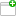This comment was attached:

arxcrash-1373-1278209285
#4
Comment posted byCrashBot
Apr 16, 14:49
A file was uploaded. maps.txtThis comment was attached:

arxcrash-1373-1278209285
#3
Comment posted byCrashBot
Apr 16, 14:49
A file was uploaded. cfg.iniThis comment was attached:

arxcrash-1373-1278209285
#1
Comment posted byCrashBot
Apr 16, 14:49
A file was uploaded. arx.logThis comment was attached:

arxcrash-1373-1278209285